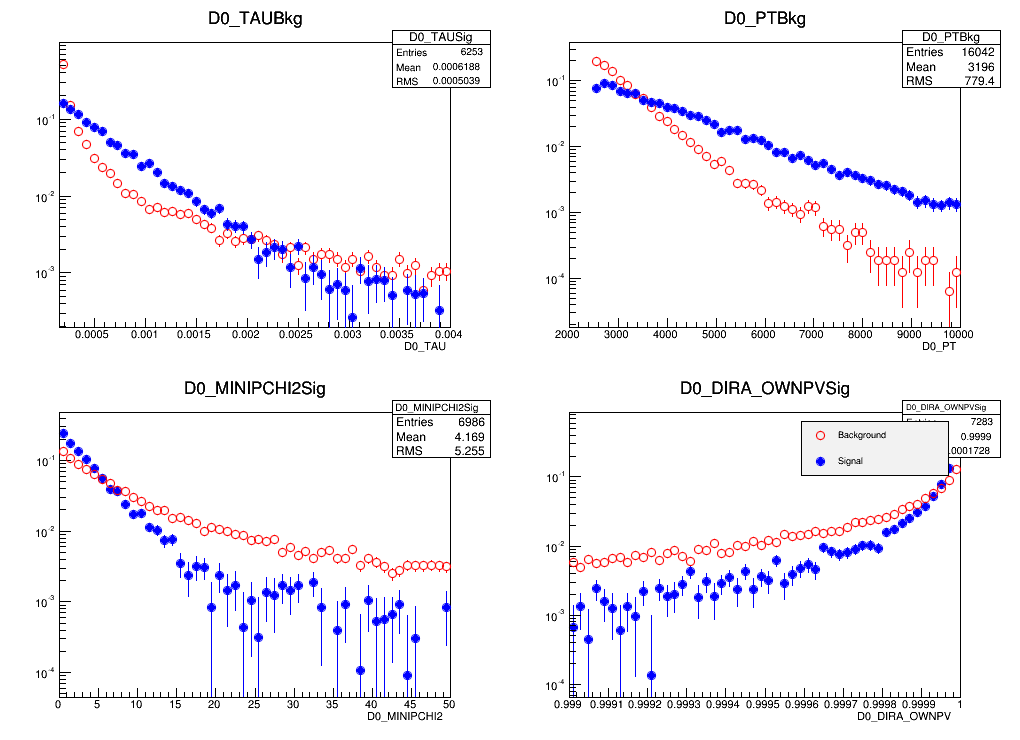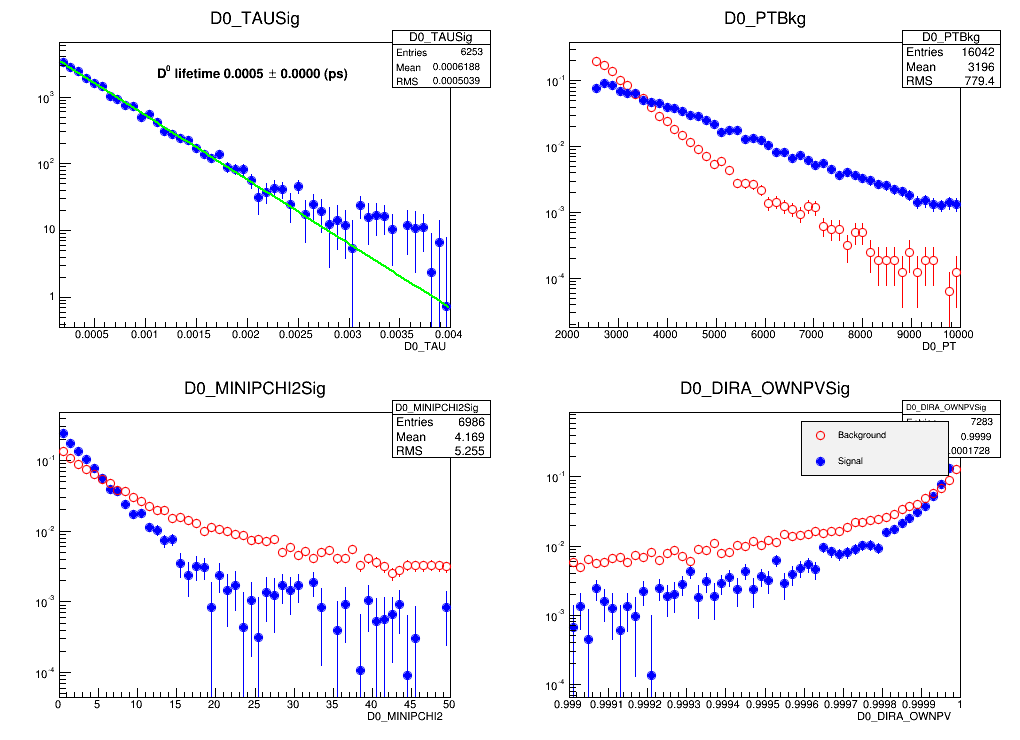## Exercise 2 : obtaining signal variable distributions¶

The object of this exercise is to use the technique of background subtraction in order to obtain the signal distributions in a variable of choice. In the previous exercise, you finished by definining a signal region, in which the events were a mixture of signal and background, and a sideband region of pure background events. You can now obtain the distribution of signal events in any given variable by subtracting the distributons found in the signal and sideband regions in the correct proportion.

1. Calculate the correct proportion for the subtraction using the background yields in the signal region and sideband region.
2. For each of the variables listed in the Introduction (D0_PT, D0_TAU, D0_DIRA_OPWNPV, D0_MINIPCHI2), plot the background subtracted signal distributions. Overplot the background distributions from the sideband region on the same canvas, in order to easily compare the two. If the signal and background distributions look very similar to each other in any variable, plot that variable on a logarithmic scale to bring out the differences.
3. Discuss these distributions with an instructor.
In :
from ROOT import TFile, RooRealVar, RooDataSet, RooArgSet, RooGaussian, RooChebychev, RooArgList, RooAddPdf, RooFit, TH1F
import rootnotes

In :
inputfile = TFile("TESHEP_Pedagogical_Data.root")
inputchain = inputfile.Get("DecayTree")

#Define the variables to be plotted and their upper and lower ranges
vars = {"D0_PT" 	: [2000.	, 10000.],
"D0_DIRA_OWNPV" : [0.999	, 1.	],
"D0_TAU" 	: [0.00015	, 0.004	],
"D0_MINIPCHI2"  : [0.   	, 50.	]}

In :
#Define the mass variable
nameofmassvar = "D0_MM"
#Define the signal range (from output of previous script)
sigrangelow  = 1849.
sigrangehigh = 1880.
#Define the sideband range to be used for the background extraction
backgroundrangelow  = 1890.
backgroundrangehigh = 1914.
#Define the background scale factor (from output of previous script)
backscale = 1.33

In :
#Define the histograms -- for each variable, one for signal and one
#for background
histos = {"sig" : {}, "bkg" : {}}
for var in vars :
histos["sig"][var] = TH1F(var+"Sig",var+"Sig",50,vars[var],vars[var])
histos["sig"][var].Sumw2() #Tell the histogram it should keep the errors on each point
histos["sig"][var].SetMarkerColor(4) #...background is blue
histos["sig"][var].SetLineColor(4)
histos["sig"][var].SetMarkerStyle(20)
histos["sig"][var].GetXaxis().SetTitle(var)
histos["bkg"][var] = TH1F(var+"Bkg",var+"Bkg",50,vars[var],vars[var])
histos["bkg"][var].Sumw2() #Tell the histogram it should keep the errors on each point
histos["bkg"][var].SetMarkerColor(2) #Signal is red...
histos["bkg"][var].SetLineColor(2)
histos["bkg"][var].SetMarkerStyle(24)
histos["bkg"][var].GetXaxis().SetTitle(var)

In :
#Fill the histograms depending on whether the mass of the event
#is in the signal or the background ranges
for entry in range(0,inputchain.GetEntries()) :
inputchain.GetEntry(entry)
tofill = ""
#If I am in the signal box fill the signal histograms
if inputchain.__getattr__(nameofmassvar) > sigrangelow and \
inputchain.__getattr__(nameofmassvar) < sigrangehigh :
tofill = "sig"
#Else if I am in the sideband fill the background histograms
elif inputchain.__getattr__(nameofmassvar) > backgroundrangelow and \
inputchain.__getattr__(nameofmassvar) < backgroundrangehigh :
tofill = "bkg"
#Else fill neither and move to the next event
else :
continue
for var in vars :
histos[tofill][var].Fill(inputchain.__getattr__(var))

In :
#Subtract the background histograms from the signal ones corrected by the scale factor
for var in vars :

In :
#Define a canvas on which to plot all of this. What we want to do is plot the
#background subtracted signal distributions and the pure background distributions
#normalized on top of each other.
mycanvas = rootnotes.default_canvas(name="c5", size=(1024, 768))

from ROOT import TLegend
mycanvas.Divide(2,2)

In :
currentpad = 1
for var in vars :
#Which is taller?
topbkg =  histos["bkg"][var].GetBinContent(histos["bkg"][var].GetMaximumBin())
topsig =  histos["sig"][var].GetBinContent(histos["sig"][var].GetMaximumBin())
if (topbkg > topsig) :
histos["bkg"][var].DrawNormalized("E")
histos["sig"][var].DrawNormalized("ESAME")
else :
histos["sig"][var].DrawNormalized("E")
histos["bkg"][var].DrawNormalized("ESAME")
#Set to log scale
legend = TLegend(0.575,0.725,0.875,0.875)
legend.SetTextSize(0.03);
legend.Draw()

In :
mycanvas

Out:## Exercise 3 : measuring the D0 lifetime¶

The objective of this exercise is to use the signal sample which you obtained in the previous step to measure the lifetime of the $D^0$ meson. This is the same quantity as the half-life of a radioactive particle : the $D^0$ decays according to an exponential distribution, and if this exponential is fitted to a distribution of the $D^0$ decay times, the slope of this exponential is the lifetime of the $D^0$.

In :
from ROOT import TF1, TLatex, kGreen, kBlack, kTRUE, Form

In :
mycanvas.cd(1);
fFitExpo = TF1("fitExpo","expo(0)",0, 30);

In :
fFitExpo.SetLineColor(kGreen);
fFitExpo.SetLineWidth(2);

In :
histos["sig"]["D0_TAU"].Fit(fFitExpo,"rmeh")
labels2 = TLatex(0,0,"Fit Parameters");
labels2.SetTextSize(0.04);
labels2.SetTextColor(kBlack);
labels2.SetNDC(kTRUE);
labels2.DrawLatex(
0.3,
0.8,
Form(
"D^{0} lifetime %.4f #pm %.4f (ps)" % (
-1./fFitExpo.GetParameter(1),
fFitExpo.GetParError(1)/(fFitExpo.GetParameter(1) * fFitExpo.GetParameter(1))
)
)
)

Out:
<ROOT.TLatex object at 0x5295e10>
In :
mycanvas.Update()

In :
mycanvas

Out:Compare obtained value with one specified by Particle Data Group.

How would you calculate a half-time of $D^{0}$?